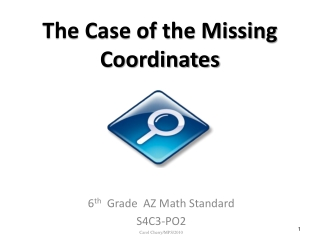DownloadDownload PresentationThe Case of the Missing Coordinates

# The Case of the Missing Coordinates

Télécharger la présentation## The Case of the Missing Coordinates

- - - - - - - - - - - - - - - - - - - - - - - - - - - E N D - - - - - - - - - - - - - - - - - - - - - - - - - - -
##### Presentation Transcript

1. The Case of the Missing Coordinates 6th Grade AZ Math Standard S4C3-PO2 Carol Cherry/MPS/2010

2. Just the Facts To help you solve the case, we’ll review some basic facts about coordinates in the next few slides.

3. Ordered Pairs Always start at the origin (0,0) The first number tells how many units to go on the horizontal, or x-axis. Right 3 units If the first number is positive, e.g. (3,4), go Left 3 units If the first number is negative, e.g. (-3,4) go

4. Up 4 units If the second number is positive, e.g. (3,4), go The second number tells how many units to go on the vertical, or y-axis. Down 4 units If the second number is negative, e.g. (3,-4), go

5. Properties of PolygonsTo solve the mystery of the missing coordinates, you need to know the properties of some important polygons.

6. Clues that a Polygon is a Square 4 Number of sides: 2 Number of pairs of parallel sides: 4 Number of right angles: 4 Number of congruent sides:

7. Clues that a Polygon is a Rectangle Number of sides: 4 2 Number of pairs of parallel sides: Number of right angles: 4 Number of congruent sides: 2 pairs of opposite sides congruent

8. Clues that a Polygon is a Parallelogram 4 Number of sides: Number of pairs of parallel sides: 2 Number of right angles: 0 Number of congruent sides: 2 pairs opposite sides congruent

9. Clues that a Polygon is an Isosceles Trapezoid Number of sides: 4 Number of pairs of parallel sides: 1 Number of right angles: 0 Number of congruent sides: One pair of opposite sides congruent

10. Example of How a Great Detective Would Solve this Case Goal: Find the missing coordinates of this parallelogram and justify your answer. Properties to use as clues: Both pairs of sides are parallel, so the bottom line, or base, has to be parallel to the top line. That means the vertex will be on the same line as the vertex at (-6,1) so 1 is the second coordinate. The base has to be congruent to the top line, which is 6 units long. So start at the left vertex, -6, and go 6 units right. The X, or first coordinate is at X=0. The missing coordinates are (0,1)

11. Now you’re ready to find some missing coordinates!

12. What are the missing coordinates of this square? (-1,-1) The coordinates are: Explain how you found them.

13. Find the coordinates for the fourth vertex of this rectangle. (-6,4) Ordered pair: Explain how you found them.

14. Locate the missing coordinates in this parallelogram See the next slide for a tip!

15. Think of the parallelogram as a rectangle and 2 triangles • The triangles are congruent • The left triangle has a base of 3 units • Therefore, the base of the triangle on the right is 3 units Now you can find the missing coordinates: (6,7)

16. Find the coordinates for the missing vertex of this parallelogram. (5,-2) Coordinates: Justify your answer.

17. Think of this isosceles trapezoid as a square plus 2 triangles. Find the missing coordinates. Missing coordinates: (5,-1)

18. One last chance to practice:Find the ordered pair for the missing vertex in this parallelogram. Tell your partner which properties of a parallelogram helped you find your answer.Ordered pair for the missing vertex: (4,7)

19. For more practice with ordered pairs, try these websites: http://www.oswego.org/ocsd-web/games/BillyBug2/bug2.html http://www.shodor.org/interactivate/activities/MazeGame/ http://funbasedlearning.com/algebra/graphing/default.htm

20. Thank you for helping solve The Case of the Missing Coordinates. Now you are ready to solve your own cases!